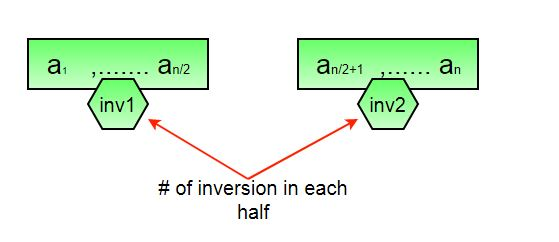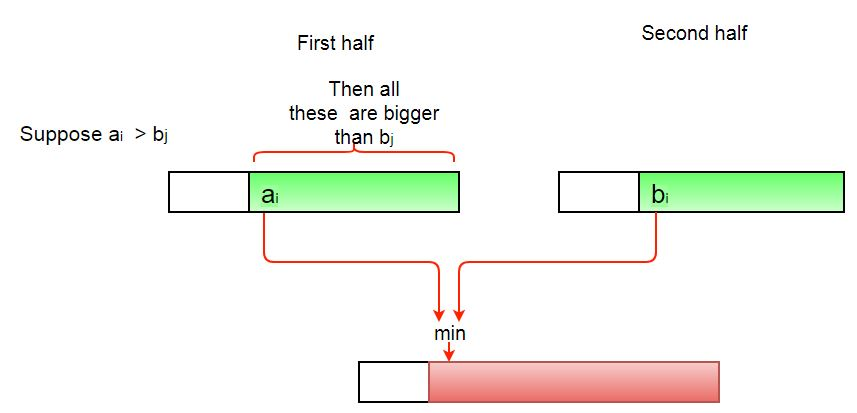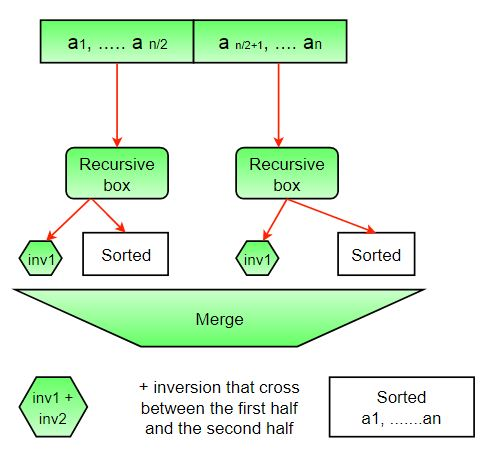# Count Inversions in an array | Set 1 (Using Merge Sort)

• Difficulty Level : Hard
• Last Updated : 20 Apr, 2022

Inversion Count for an array indicates – how far (or close) the array is from being sorted. If the array is already sorted, then the inversion count is 0, but if the array is sorted in the reverse order, the inversion count is the maximum.
Formally speaking, two elements a[i] and a[j] form an inversion if a[i] > a[j] and i < j
Example:

```Input: arr[] = {8, 4, 2, 1}
Output: 6

Explanation: Given array has six inversions:
(8, 4), (4, 2), (8, 2), (8, 1), (4, 1), (2, 1).

Input: arr[] = {3, 1, 2}
Output: 2

Explanation: Given array has two inversions:
(3, 1), (3, 2) ```

METHOD 1 (Simple)

• Approach: Traverse through the array, and for every index, find the number of smaller elements on its right side of the array. This can be done using a nested loop. Sum up the counts for all index in the array and print the sum.
• Algorithm:
1. Traverse through the array from start to end
2. For every element, find the count of elements smaller than the current number up to that index using another loop.
3. Sum up the count of inversion for every index.
4. Print the count of inversions.
• Implementation:

## C++

 `// C++ program to Count Inversions``// in an array``#include ``using` `namespace` `std;` `int` `getInvCount(``int` `arr[], ``int` `n)``{``    ``int` `inv_count = 0;``    ``for` `(``int` `i = 0; i < n - 1; i++)``        ``for` `(``int` `j = i + 1; j < n; j++)``            ``if` `(arr[i] > arr[j])``                ``inv_count++;` `    ``return` `inv_count;``}` `// Driver Code``int` `main()``{``    ``int` `arr[] = { 1, 20, 6, 4, 5 };``    ``int` `n = ``sizeof``(arr) / ``sizeof``(arr);``    ``cout << ``" Number of inversions are "``         ``<< getInvCount(arr, n);``    ``return` `0;``}` `// This code is contributed``// by Akanksha Rai`

## C

 `// C program to Count``// Inversions in an array``#include ``#include ``int` `getInvCount(``int` `arr[], ``int` `n)``{``    ``int` `inv_count = 0;``    ``for` `(``int` `i = 0; i < n - 1; i++)``        ``for` `(``int` `j = i + 1; j < n; j++)``            ``if` `(arr[i] > arr[j])``                ``inv_count++;` `    ``return` `inv_count;``}` `/* Driver program to test above functions */``int` `main()``{``    ``int` `arr[] = { 1, 20, 6, 4, 5 };``    ``int` `n = ``sizeof``(arr) / ``sizeof``(arr);``    ``printf``(``" Number of inversions are %d \n"``,``           ``getInvCount(arr, n));``    ``return` `0;``}`

## Java

 `// Java program to count``// inversions in an array``class` `Test {``    ``static` `int` `arr[] = ``new` `int``[] { ``1``, ``20``, ``6``, ``4``, ``5` `};` `    ``static` `int` `getInvCount(``int` `n)``    ``{``        ``int` `inv_count = ``0``;``        ``for` `(``int` `i = ``0``; i < n - ``1``; i++)``            ``for` `(``int` `j = i + ``1``; j < n; j++)``                ``if` `(arr[i] > arr[j])``                    ``inv_count++;` `        ``return` `inv_count;``    ``}` `    ``// Driver method to test the above function``    ``public` `static` `void` `main(String[] args)``    ``{``        ``System.out.println(``"Number of inversions are "``                           ``+ getInvCount(arr.length));``    ``}``}`

## Python3

 `# Python3 program to count``# inversions in an array`  `def` `getInvCount(arr, n):` `    ``inv_count ``=` `0``    ``for` `i ``in` `range``(n):``        ``for` `j ``in` `range``(i ``+` `1``, n):``            ``if` `(arr[i] > arr[j]):``                ``inv_count ``+``=` `1` `    ``return` `inv_count`  `# Driver Code``arr ``=` `[``1``, ``20``, ``6``, ``4``, ``5``]``n ``=` `len``(arr)``print``(``"Number of inversions are"``,``      ``getInvCount(arr, n))` `# This code is contributed by Smitha Dinesh Semwal`

## C#

 `// C# program to count inversions``// in an array``using` `System;``using` `System.Collections.Generic;` `class` `GFG {` `    ``static` `int``[] arr = ``new` `int``[] { 1, 20, 6, 4, 5 };` `    ``static` `int` `getInvCount(``int` `n)``    ``{``        ``int` `inv_count = 0;` `        ``for` `(``int` `i = 0; i < n - 1; i++)``            ``for` `(``int` `j = i + 1; j < n; j++)``                ``if` `(arr[i] > arr[j])``                    ``inv_count++;` `        ``return` `inv_count;``    ``}` `    ``// Driver code``    ``public` `static` `void` `Main()``    ``{``        ``Console.WriteLine(``"Number of "``                          ``+ ``"inversions are "``                          ``+ getInvCount(arr.Length));``    ``}``}` `// This code is contributed by Sam007`

## PHP

 ` ``\$arr``[``\$j``])``                ``\$inv_count``++;` `    ``return` `\$inv_count``;``}` `// Driver Code``\$arr` `= ``array``(1, 20, 6, 4, 5 );``\$n` `= sizeof(``\$arr``);``echo` `"Number of inversions are "``,``           ``getInvCount(``\$arr``, ``\$n``);` `// This code is contributed by ita_c``?>`

## Javascript

 ``
Output
` Number of inversions are 5`
• Complexity Analysis:
• Time Complexity: O(n^2), Two nested loops are needed to traverse the array from start to end, so the Time complexity is O(n^2)
• Space Complexity:O(1), No extra space is required.

METHOD 2(Enhance Merge Sort)

• Approach:
Suppose the number of inversions in the left half and right half of the array (let be inv1 and inv2); what kinds of inversions are not accounted for in Inv1 + Inv2? The answer is – the inversions that need to be counted during the merge step. Therefore, to get the total number of inversions that needs to be added are the number of inversions in the left subarray, right subarray, and merge().• How to get the number of inversions in merge()?
In merge process, let i is used for indexing left sub-array and j for right sub-array. At any step in merge(), if a[i] is greater than a[j], then there are (mid – i) inversions. because left and right subarrays are sorted, so all the remaining elements in left-subarray (a[i+1], a[i+2] … a[mid]) will be greater than a[j]• The complete picture:• Algorithm:
1. The idea is similar to merge sort, divide the array into two equal or almost equal halves in each step until the base case is reached.
2. Create a function merge that counts the number of inversions when two halves of the array are merged, create two indices i and j, i is the index for the first half, and j is an index of the second half. if a[i] is greater than a[j], then there are (mid – i) inversions. because left and right subarrays are sorted, so all the remaining elements in left-subarray (a[i+1], a[i+2] … a[mid]) will be greater than a[j].
3. Create a recursive function to divide the array into halves and find the answer by summing the number of inversions is the first half, the number of inversion in the second half and the number of inversions by merging the two.
4. The base case of recursion is when there is only one element in the given half.
• Implementation:

## C++

 `// C++ program to Count``// Inversions in an array``// using Merge Sort``#include ``using` `namespace` `std;` `int` `_mergeSort(``int` `arr[], ``int` `temp[], ``int` `left, ``int` `right);``int` `merge(``int` `arr[], ``int` `temp[], ``int` `left, ``int` `mid,``          ``int` `right);` `/* This function sorts the``   ``input array and returns the``number of inversions in the array */``int` `mergeSort(``int` `arr[], ``int` `array_size)``{``    ``int` `temp[array_size];``    ``return` `_mergeSort(arr, temp, 0, array_size - 1);``}` `/* An auxiliary recursive function``  ``that sorts the input array and``returns the number of inversions in the array. */``int` `_mergeSort(``int` `arr[], ``int` `temp[], ``int` `left, ``int` `right)``{``    ``int` `mid, inv_count = 0;``    ``if` `(right > left) {``        ``/* Divide the array into two parts and``        ``call _mergeSortAndCountInv()``        ``for each of the parts */``        ``mid = (right + left) / 2;` `        ``/* Inversion count will be sum of``        ``inversions in left-part, right-part``        ``and number of inversions in merging */``        ``inv_count += _mergeSort(arr, temp, left, mid);``        ``inv_count += _mergeSort(arr, temp, mid + 1, right);` `        ``/*Merge the two parts*/``        ``inv_count += merge(arr, temp, left, mid + 1, right);``    ``}``    ``return` `inv_count;``}` `/* This function merges two sorted arrays``and returns inversion count in the arrays.*/``int` `merge(``int` `arr[], ``int` `temp[], ``int` `left, ``int` `mid,``          ``int` `right)``{``    ``int` `i, j, k;``    ``int` `inv_count = 0;` `    ``i = left; ``/* i is index for left subarray*/``    ``j = mid; ``/* j is index for right subarray*/``    ``k = left; ``/* k is index for resultant merged subarray*/``    ``while` `((i <= mid - 1) && (j <= right)) {``        ``if` `(arr[i] <= arr[j]) {``            ``temp[k++] = arr[i++];``        ``}``        ``else` `{``            ``temp[k++] = arr[j++];` `            ``/* this is tricky -- see above``            ``explanation/diagram for merge()*/``            ``inv_count = inv_count + (mid - i);``        ``}``    ``}` `    ``/* Copy the remaining elements of left subarray``(if there are any) to temp*/``    ``while` `(i <= mid - 1)``        ``temp[k++] = arr[i++];` `    ``/* Copy the remaining elements of right subarray``       ``(if there are any) to temp*/``    ``while` `(j <= right)``        ``temp[k++] = arr[j++];` `    ``/*Copy back the merged elements to original array*/``    ``for` `(i = left; i <= right; i++)``        ``arr[i] = temp[i];` `    ``return` `inv_count;``}` `// Driver code``int` `main()``{``    ``int` `arr[] = { 1, 20, 6, 4, 5 };``    ``int` `n = ``sizeof``(arr) / ``sizeof``(arr);``    ``int` `ans = mergeSort(arr, n);``    ``cout << ``" Number of inversions are "` `<< ans;``    ``return` `0;``}` `// This is code is contributed by rathbhupendra`

## C

 `// C program to Count``// Inversions in an array``// using Merge Sort``#include ``#include ` `int` `_mergeSort(``int` `arr[], ``int` `temp[], ``int` `left, ``int` `right);``int` `merge(``int` `arr[], ``int` `temp[], ``int` `left, ``int` `mid,``          ``int` `right);` `/* This function sorts the input array and returns the``   ``number of inversions in the array */``int` `mergeSort(``int` `arr[], ``int` `array_size)``{``    ``int``* temp = (``int``*)``malloc``(``sizeof``(``int``) * array_size);``    ``return` `_mergeSort(arr, temp, 0, array_size - 1);``}` `/* An auxiliary recursive function``   ``that sorts the input``  ``array and returns the number``  ``of inversions in the array.``*/``int` `_mergeSort(``int` `arr[], ``int` `temp[], ``int` `left, ``int` `right)``{``    ``int` `mid, inv_count = 0;``    ``if` `(right > left) {``        ``/* Divide the array into two parts and call``       ``_mergeSortAndCountInv() for each of the parts */``        ``mid = (right + left) / 2;` `        ``/* Inversion count will be the sum of inversions in``        ``left-part, right-part and number of inversions in``        ``merging */``        ``inv_count += _mergeSort(arr, temp, left, mid);``        ``inv_count += _mergeSort(arr, temp, mid + 1, right);` `        ``/*Merge the two parts*/``        ``inv_count += merge(arr, temp, left, mid + 1, right);``    ``}``    ``return` `inv_count;``}` `/* This function merges two sorted``   ``arrays and returns inversion``   ``count in the arrays.*/``int` `merge(``int` `arr[], ``int` `temp[], ``int` `left, ``int` `mid,``          ``int` `right)``{``    ``int` `i, j, k;``    ``int` `inv_count = 0;` `    ``i = left; ``/* i is index for left subarray*/``    ``j = mid; ``/* j is index for right subarray*/``    ``k = left; ``/* k is index for resultant merged subarray*/``    ``while` `((i <= mid - 1) && (j <= right)) {``        ``if` `(arr[i] <= arr[j]) {``            ``temp[k++] = arr[i++];``        ``}``        ``else` `{``            ``temp[k++] = arr[j++];` `            ``/*this is tricky -- see above``             ``* explanation/diagram for merge()*/``            ``inv_count = inv_count + (mid - i);``        ``}``    ``}` `    ``/* Copy the remaining elements of left subarray``   ``(if there are any) to temp*/``    ``while` `(i <= mid - 1)``        ``temp[k++] = arr[i++];` `    ``/* Copy the remaining elements of right subarray``   ``(if there are any) to temp*/``    ``while` `(j <= right)``        ``temp[k++] = arr[j++];` `    ``/*Copy back the merged elements to original array*/``    ``for` `(i = left; i <= right; i++)``        ``arr[i] = temp[i];` `    ``return` `inv_count;``}` `/* Driver code*/``int` `main(``int` `argv, ``char``** args)``{``    ``int` `arr[] = { 1, 20, 6, 4, 5 };``    ``printf``(``" Number of inversions are %d \n"``,``           ``mergeSort(arr, 5));``    ``getchar``();``    ``return` `0;``}`

## Java

 `// Java implementation of the approach``import` `java.util.Arrays;` `public` `class` `GFG {` `    ``// Function to count the number of inversions``    ``// during the merge process``    ``private` `static` `int` `mergeAndCount(``int``[] arr, ``int` `l,``                                     ``int` `m, ``int` `r)``    ``{` `        ``// Left subarray``        ``int``[] left = Arrays.copyOfRange(arr, l, m + ``1``);` `        ``// Right subarray``        ``int``[] right = Arrays.copyOfRange(arr, m + ``1``, r + ``1``);` `        ``int` `i = ``0``, j = ``0``, k = l, swaps = ``0``;` `        ``while` `(i < left.length && j < right.length) {``            ``if` `(left[i] <= right[j])``                ``arr[k++] = left[i++];``            ``else` `{``                ``arr[k++] = right[j++];``                ``swaps += (m + ``1``) - (l + i);``            ``}``        ``}``        ``while` `(i < left.length)``            ``arr[k++] = left[i++];``        ``while` `(j < right.length)``            ``arr[k++] = right[j++];``        ``return` `swaps;``    ``}` `    ``// Merge sort function``    ``private` `static` `int` `mergeSortAndCount(``int``[] arr, ``int` `l,``                                         ``int` `r)``    ``{` `        ``// Keeps track of the inversion count at a``        ``// particular node of the recursion tree``        ``int` `count = ``0``;` `        ``if` `(l < r) {``            ``int` `m = (l + r) / ``2``;` `            ``// Total inversion count = left subarray count``            ``// + right subarray count + merge count` `            ``// Left subarray count``            ``count += mergeSortAndCount(arr, l, m);` `            ``// Right subarray count``            ``count += mergeSortAndCount(arr, m + ``1``, r);` `            ``// Merge count``            ``count += mergeAndCount(arr, l, m, r);``        ``}` `        ``return` `count;``    ``}` `    ``// Driver code``    ``public` `static` `void` `main(String[] args)``    ``{``        ``int``[] arr = { ``1``, ``20``, ``6``, ``4``, ``5` `};` `        ``System.out.println(``            ``mergeSortAndCount(arr, ``0``, arr.length - ``1``));``    ``}``}` `// This code is contributed by Pradip Basak`

## Python3

 `# Python 3 program to count inversions in an array` `# Function to Use Inversion Count`  `def` `mergeSort(arr, n):``    ``# A temp_arr is created to store``    ``# sorted array in merge function``    ``temp_arr ``=` `[``0``]``*``n``    ``return` `_mergeSort(arr, temp_arr, ``0``, n``-``1``)` `# This Function will use MergeSort to count inversions`  `def` `_mergeSort(arr, temp_arr, left, right):` `    ``# A variable inv_count is used to store``    ``# inversion counts in each recursive call` `    ``inv_count ``=` `0` `    ``# We will make a recursive call if and only if``    ``# we have more than one elements` `    ``if` `left < right:` `        ``# mid is calculated to divide the array into two subarrays``        ``# Floor division is must in case of python` `        ``mid ``=` `(left ``+` `right)``/``/``2` `        ``# It will calculate inversion``        ``# counts in the left subarray` `        ``inv_count ``+``=` `_mergeSort(arr, temp_arr,``                                ``left, mid)` `        ``# It will calculate inversion``        ``# counts in right subarray` `        ``inv_count ``+``=` `_mergeSort(arr, temp_arr,``                                ``mid ``+` `1``, right)` `        ``# It will merge two subarrays in``        ``# a sorted subarray` `        ``inv_count ``+``=` `merge(arr, temp_arr, left, mid, right)``    ``return` `inv_count` `# This function will merge two subarrays``# in a single sorted subarray`  `def` `merge(arr, temp_arr, left, mid, right):``    ``i ``=` `left     ``# Starting index of left subarray``    ``j ``=` `mid ``+` `1`  `# Starting index of right subarray``    ``k ``=` `left     ``# Starting index of to be sorted subarray``    ``inv_count ``=` `0` `    ``# Conditions are checked to make sure that``    ``# i and j don't exceed their``    ``# subarray limits.` `    ``while` `i <``=` `mid ``and` `j <``=` `right:` `        ``# There will be no inversion if arr[i] <= arr[j]` `        ``if` `arr[i] <``=` `arr[j]:``            ``temp_arr[k] ``=` `arr[i]``            ``k ``+``=` `1``            ``i ``+``=` `1``        ``else``:``            ``# Inversion will occur.``            ``temp_arr[k] ``=` `arr[j]``            ``inv_count ``+``=` `(mid``-``i ``+` `1``)``            ``k ``+``=` `1``            ``j ``+``=` `1` `    ``# Copy the remaining elements of left``    ``# subarray into temporary array``    ``while` `i <``=` `mid:``        ``temp_arr[k] ``=` `arr[i]``        ``k ``+``=` `1``        ``i ``+``=` `1` `    ``# Copy the remaining elements of right``    ``# subarray into temporary array``    ``while` `j <``=` `right:``        ``temp_arr[k] ``=` `arr[j]``        ``k ``+``=` `1``        ``j ``+``=` `1` `    ``# Copy the sorted subarray into Original array``    ``for` `loop_var ``in` `range``(left, right ``+` `1``):``        ``arr[loop_var] ``=` `temp_arr[loop_var]` `    ``return` `inv_count`  `# Driver Code``# Given array is``arr ``=` `[``1``, ``20``, ``6``, ``4``, ``5``]``n ``=` `len``(arr)``result ``=` `mergeSort(arr, n)``print``(``"Number of inversions are"``, result)` `# This code is contributed by ankush_953`

## C#

 `// C# implementation of counting the``// inversion using merge sort` `using` `System;``public` `class` `Test {` `    ``/* This method sorts the input array and returns the``       ``number of inversions in the array */``    ``static` `int` `mergeSort(``int``[] arr, ``int` `array_size)``    ``{``        ``int``[] temp = ``new` `int``[array_size];``        ``return` `_mergeSort(arr, temp, 0, array_size - 1);``    ``}` `    ``/* An auxiliary recursive method that sorts the input``      ``array and returns the number of inversions in the``      ``array. */``    ``static` `int` `_mergeSort(``int``[] arr, ``int``[] temp, ``int` `left,``                          ``int` `right)``    ``{``        ``int` `mid, inv_count = 0;``        ``if` `(right > left) {``            ``/* Divide the array into two parts and call``           ``_mergeSortAndCountInv() for each of the parts */``            ``mid = (right + left) / 2;` `            ``/* Inversion count will be the sum of inversions``          ``in left-part, right-part``          ``and number of inversions in merging */``            ``inv_count += _mergeSort(arr, temp, left, mid);``            ``inv_count``                ``+= _mergeSort(arr, temp, mid + 1, right);` `            ``/*Merge the two parts*/``            ``inv_count``                ``+= merge(arr, temp, left, mid + 1, right);``        ``}``        ``return` `inv_count;``    ``}` `    ``/* This method merges two sorted arrays and returns``       ``inversion count in the arrays.*/``    ``static` `int` `merge(``int``[] arr, ``int``[] temp, ``int` `left,``                     ``int` `mid, ``int` `right)``    ``{``        ``int` `i, j, k;``        ``int` `inv_count = 0;` `        ``i = left; ``/* i is index for left subarray*/``        ``j = mid; ``/* j is index for right subarray*/``        ``k = left; ``/* k is index for resultant merged``                     ``subarray*/``        ``while` `((i <= mid - 1) && (j <= right)) {``            ``if` `(arr[i] <= arr[j]) {``                ``temp[k++] = arr[i++];``            ``}``            ``else` `{``                ``temp[k++] = arr[j++];` `                ``/*this is tricky -- see above``                 ``* explanation/diagram for merge()*/``                ``inv_count = inv_count + (mid - i);``            ``}``        ``}` `        ``/* Copy the remaining elements of left subarray``       ``(if there are any) to temp*/``        ``while` `(i <= mid - 1)``            ``temp[k++] = arr[i++];` `        ``/* Copy the remaining elements of right subarray``       ``(if there are any) to temp*/``        ``while` `(j <= right)``            ``temp[k++] = arr[j++];` `        ``/*Copy back the merged elements to original array*/``        ``for` `(i = left; i <= right; i++)``            ``arr[i] = temp[i];` `        ``return` `inv_count;``    ``}` `    ``// Driver method to test the above function``    ``public` `static` `void` `Main()``    ``{``        ``int``[] arr = ``new` `int``[] { 1, 20, 6, 4, 5 };``        ``Console.Write(``"Number of inversions are "``                      ``+ mergeSort(arr, 5));``    ``}``}``// This code is contributed by Rajput-Ji`

## Javascript

 ``

Output:

`Number of inversions are 5`

Complexity Analysis:

• Time Complexity: O(n log n), The algorithm used is divide and conquer, So in each level, one full array traversal is needed, and there are log n levels, so the time complexity is O(n log n).
• Space Complexity: O(n), Temporary array.

Note that the above code modifies (or sorts) the input array. If we want to count only inversions, we need to create a copy of the original array and call mergeSort() on the copy to preserve the original array’s order.

METHOD 3(Heapsort + Bisection)

• Algorithm:
1. Create a heap with new pair elements,  (element, index).
2. After sorting them, pop out each minimum sequently and create a new sorted list with the indexes. 3. Calculate the difference between the original index and the index of bisection of the new sorted list.
4. Sum up the difference.

## Python3

 `from` `heapq ``import` `heappush, heappop``from` `bisect ``import` `bisect, insort`  `def` `getNumOfInversions(A):``    ``N ``=` `len``(A)``    ``if` `N <``=` `1``:``        ``return` `0` `    ``sortList ``=` `[]``    ``result ``=` `0` `    ``# heapsort, O(N*log(N))``    ``for` `i, v ``in` `enumerate``(A):``        ``heappush(sortList, (v, i))` `    ``x ``=` `[]  ``# create a sorted list of indexes``    ``while` `sortList:  ``# O(N)``        ``v, i ``=` `heappop(sortList)  ``# O(log(N))``        ``# find the current minimum's index``        ``# the index y can represent how many minimums on the left``        ``y ``=` `bisect(x, i)  ``# O(log(N))``        ``# i can represent how many elements on the left``        ``# i - y can find how many bigger nums on the left``        ``result ``+``=` `i ``-` `y` `        ``insort(x, i)  ``# O(log(N))` `    ``return` `result` `# Driver Code``# Given array is``A ``=` `[``-``1``, ``6``, ``3``, ``4``, ``7``, ``4``]``result ``=` `getNumOfInversions(A)``print``(f``'Number of inversions are {result}'``)`

Complexity Analysis:

• Time Complexity: O(n log n). Both heapsort and bisection can perform sorted insertion in log n in each element, so the time complexity is O(n log n).
• Space Complexity: O(n). A heap and a new list are the same length as the original array.

You may like to see:
Count inversions in an array | Set 2 (Using Self-Balancing BST)
Counting Inversions using Set in C++ STL
Count inversions in an array | Set 3 (Using BIT)
References:
http://www.cs.umd.edu/class/fall2009/cmsc451/lectures/Lec08-inversions.pdf
http://www.cp.eng.chula.ac.th/~piak/teaching/algo/algo2008/count-inv.htm
Please write comments if you find any bug in the above program/algorithm or other ways to solve it.

My Personal Notes arrow_drop_up